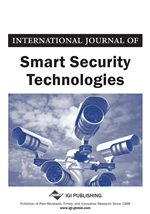# Distributed Quantiles Estimation of Sensor Network Measurements

Jongmin Lee, Cihan Tepedelenlioglu, Andreas Spanias, Gowtham Muniraju
DOI: 10.4018/IJSST.2020070103
OnDemand:
(Individual Articles)
Available
\$37.50
No Current Special Offers

## Abstract

A quantile is a value below which random draws from a distribution falls with a given probability. In a centralized setting where the cumulative distribution function (CDF) is unknown, empirical CDF can be used to estimate quantiles after data aggregation. In a distributed sensor network, it is challenging to estimate quantiles, as each sensor observes local measurement data with limited storage and transmission power, which makes it difficult to obtain the global ECDF. This paper proposes consensus-based quantile estimation for such networks, even when communication links are corrupted by independent random noise. The state-values are recursively updated with two steps: a local update based on measurement data and current state and averaging updated states with local nodes. The estimated state sequence is shown to be asymptotically unbiased and converges toward the sample quantile in the mean-square sense. Applications on the distributed estimation of trimmed mean; computation of median, maximum, or minimum values; and identification of outliers through simulation are also provided.
Article Preview
Top

## Introduction

Distributed sensors measure physical phenomena observable over a certain region and fuse the sensed information by local communications. This type of network is scalable and energy efficient because each node shares its data only with neighbors. A traditional problem in this domain is to estimate the sample average of measurements by iteratively averaging the states with neighboring ones, and achieve a consensus on the global average of the initial measurements (Xiao and Boyd, 2004; Xiao et al. 2005, Olfati-Saber et al., 2007). This has influenced many distributed estimation applications due to the broad use of the arithmetic mean in signal processing techniques.

Distributed average consensus of sensor measurement data can be used in monitoring applications (Muniraju et al, 2020). One example is monitoring average temperature (or, other statistical metrics) over a sensor network in remote areas. The arithmetic mean of temperature data represents the central tendency of temperature. However, the mean can be vulnerable, as a measure of central tendency, to the skewness of the distribution. Outliers can also cause bias to the sample mean. An alternative metric is the median that represents the midpoint which divides the ordered dataset into two subsets of equal size. More generally quantiles are the generalized inverse of the CDF at a certain probability. Beyond estimating the median, quantiles can be used in various applications such as outlier removal and computation of robust statistics from a set of measurement data by eliminating the values higher (or lower) than a certain cut-point. One such robust statistic is the trimmed mean which is an average of the data excluding outliers. Maximum and minimum values can be viewed as extreme examples of quantiles. Quantile regression estimates the conditional quantiles of measurement data distribution where the statistics such as mean and variance may change over time. This method has been used in a variety of machine learning (Meinshausen, 2006) as well as statistical applications (Koenker, 2005).

This paper considers the quantile estimation problem in a distributed setting, as illustrated in Figure 1. This is necessary if nodes in a distributed network have local measurement data only but want to know the quantile value without the knowledge of the global aggregate CDF estimate at each node. The sensor network is assumed to be fully distributed where there is no fusion center and the sensors are unlabeled. Each node maintains its own data and state of estimate, and communicates the information only with neighboring nodes via noisy communication links between nodes. Detailed knowledge of the network graph structure beyond neighbors is inaccessible to the nodes (Zhang et al., 2019). The states of quantile estimates are recursively updated with two steps at each iteration. The local update step is based on the individual measurement data and the current state of the quantile estimate. The updates are transferred to the neighboring nodes by averaging the estimates. This work studies the convergence behavior by showing that the estimated state sequence is asymptotically unbiased and converges toward the true quantile in the mean-square sense. The proposed algorithm is applicable for identifying outliers and calculating median as well as trimmed mean.

## Complete Article List

Search this Journal:
Reset
Volume 10: 1 Issue (2023): Forthcoming, Available for Pre-Order
Volume 9: 2 Issues (2022): 1 Released, 1 Forthcoming
Volume 8: 2 Issues (2021)
Volume 7: 2 Issues (2020)
Volume 6: 2 Issues (2019)
View Complete Journal Contents Listing